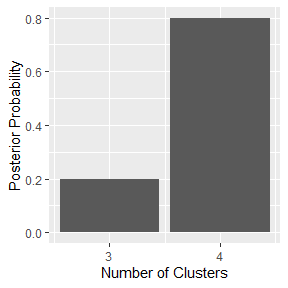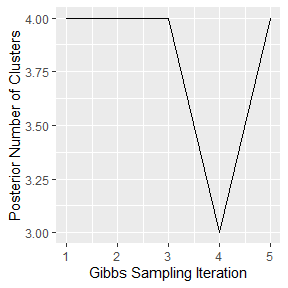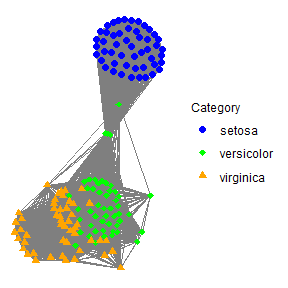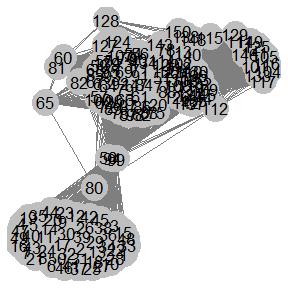# iris-NIW-vignette

library(tip)
# Import the iris dataset
data(iris)

# The first 4 columns are the data whereas
# the 5th column refers to the true labels
X <- data.matrix(iris[,c("Sepal.Length",
"Sepal.Width",
"Petal.Length",
"Petal.Width")])

# Extract the true labels (optional)
# True labels are only necessary for constructing network
# graphs that incorporate the true labels; this is often
# for research.
true_labels <- iris[,"Species"]

# Compute the distance matrix
distance_matrix <- data.matrix(dist(X))

# Compute the temperature parameter estiamte
temperature <- 1/median(distance_matrix[upper.tri(distance_matrix)])

# For each subject, compute the point estimate for the number of similar
# subjects using  univariate multiple change point detection (i.e.)
init_num_neighbors = get_cpt_neighbors(.distance_matrix = distance_matrix)

# Set the number of burn-in iterations in the Gibbs samlper
# A very good result for Iris may be obtained by setting burn <- 1000
burn <- 5

# Set the number of sampling iterations in the Gibbs sampler
# A very good result for Iris may be obtained by setting samples <- 1000
samples <- 5

# Set the subject names
names_subjects <- paste(1:dim(iris))

# Run TIP clustering using only the prior
# --> That is, the likelihood function is constant
tip1 <- tip(.data = data.matrix(X),
.burn = burn,
.samples = samples,
.similarity_matrix = exp(-1.0*temperature*distance_matrix),
.init_num_neighbors = init_num_neighbors,
.likelihood_model = "NIW",
.subject_names = names_subjects,
.num_cores = 1)
#> Bayesian Clustering: Table Invitation Prior Gibbs Sampler
#> burn-in: 5
#> samples: 5
#> Likelihood Model: NIW
#>
|
|                                                                      |   0%
|
|=========                                                             |  12%
|
|==================                                                    |  25%
|
|==========================                                            |  38%
|
|===================================                                   |  50%
|
|============================================                          |  62%
|
|====================================================                  |  75%
|
|=============================================================         |  88%
|
|======================================================================| 100%
# Produce plots for the Bayesian Clustering Model
tip_plots <- plot(tip1)
# View the posterior distribution of the number of clusters
tip_plots$histogram_posterior_number_of_clusters# View the trace plot with respect to the posterior number of clusters tip_plots$trace_plot_posterior_number_of_clusters# Extract posterior cluster assignments using the Posterior Expected Adjusted Rand (PEAR) index
cluster_assignments <- mcclust::maxpear(psm = tip1@posterior_similarity_matrix)$cl # If the true labels are available, then show the cluster result via a contigency table table(data.frame(true_label = true_labels, cluster_assignment = cluster_assignments)) #> cluster_assignment #> true_label 1 2 3 4 5 #> setosa 50 0 0 0 0 #> versicolor 0 41 2 4 3 #> virginica 0 13 37 0 0 # Create the one component graph with minimum entropy partition_list <- partition_undirected_graph(.graph_matrix = tip1@posterior_similarity_matrix, .num_components = 1, .step_size = 0.001) # Associate class labels and colors for the plot class_palette_colors <- c("setosa" = "blue", "versicolor" = 'green', "virginica" = "orange") # Associate class labels and shapes for the plot class_palette_shapes <- c("setosa" = 19, "versicolor" = 18, "virginica" = 17) # Visualize the posterior similarity matrix by constructing a graph plot of # the one-cluster graph. The true labels are used here (below they are not). ggnet2_network_plot(.matrix_graph = partition_list$partitioned_graph_matrix,
.subject_names = NA,
.subject_class_names = true_labels,
.class_colors = class_palette_colors,
.class_shapes = class_palette_shapes,
.node_size = 2,
#> Warning: Duplicated override.aes is ignored.# If true labels are not available, then construct a network plot
.add_node_labels = TRUE)